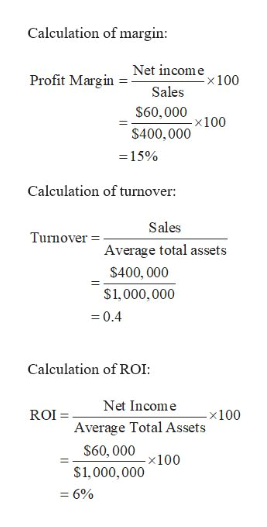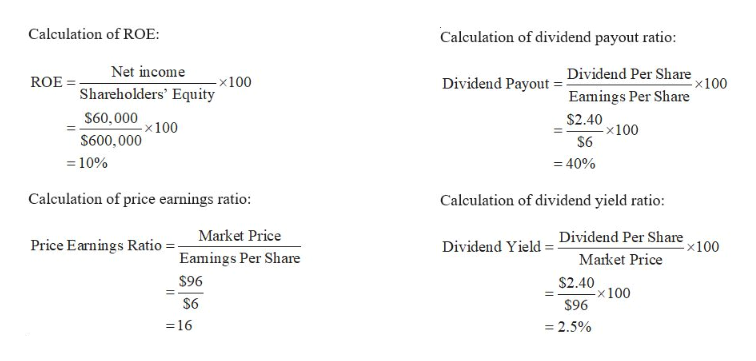# Calculate profitability measures The following information was available for the year ended December 31, 2016:Sales \$  400,000Net income 60,000Average total assets 1,000,000Average total stockholders' equity  600,000Dividends per share 2.40Earnings per share 6.00Market price per share at year-end 96.00Required:Calculate margin, turnover, and ROI for the year ended December 31, 2016.Calculate ROE for the year ended December 31, 2016.Calculate the price/earnings ratio for 2016.Calculate the dividend payout ratio for 2016.Calculate the dividend yield for 2016.

Question
46 views

Calculate profitability measures The following information was available for the year ended December 31, 2016:
Sales \$  400,000
Net income 60,000
Average total assets 1,000,000
Average total stockholders' equity  600,000
Dividends per share 2.40
Earnings per share 6.00
Market price per share at year-end 96.00
Required:
Calculate margin, turnover, and ROI for the year ended December 31, 2016.
Calculate ROE for the year ended December 31, 2016.
Calculate the price/earnings ratio for 2016.
Calculate the dividend payout ratio for 2016.
Calculate the dividend yield for 2016.

check_circle

Step 1

Margin:

Margin means the percentage of profit in the sales, it can be calculated by dividing net income by sales. This ratio is higher better.

Return on Investment (ROI):

It measures the results or efficiency of an investment. It shows the efficiency of the business investment. It is can be calculated as a ratio of net income and average total investment.

Return on equity (ROE):

It also measures the financial performance of the business in terms of return earned against the average stockholder’s equity.

Price earnings ratio:

It is calculated by dividing market price per share by earning per share. It helps to check whether or not the share is undervalued or overvalued.

Dividend payout ratio:

It is that part of earnings which is distributed as a dividend to the shareholders. Thus, to calculate dividend payout ratio, dividend per share is divided by earnings per share.

Dividend yield:

It measures the amount distributed as a dividend to the shareholders concerning the market price per share. Thus, to calculate dividend yield, dividend per share is divided by the current share price.

Step 2help_outlineImage TranscriptioncloseCalculation of margin: Profit Margin Net incom e Sales x100 \$60,000 x100 \$400,000 15% Calculation of turnover Sales Turnover Average total assets \$400, 000 S1,000, 000 0.4 Calculation of ROI: Net Income x100 Average Total Assets \$60,000x100 ROI \$1,000,000 = 6% fullscreen
Step 3help_outlineImage TranscriptioncloseCalculation of ROE Calculation of dividend payout ratio: Dividend Payout = Dividend Per Slhare x100 Eamings Per Share Net income -x 100 Shareholders' Equity \$60,000100 ROE \$2.40 -x100 \$6 \$600,000 10% -40% Calculation of price earnings ratio: Calculation of dividend yield ratio: Dividend Yield = Dividend Per Share Market Price Market Price x100 Price Earnings Ratio Eamings Per Share \$96 \$2.40 x 100 \$96 \$6 = 16 2.5% fullscreen

### Want to see the full answer?

See Solution

#### Want to see this answer and more?

Solutions are written by subject experts who are available 24/7. Questions are typically answered within 1 hour.*

See Solution
*Response times may vary by subject and question.
Tagged in